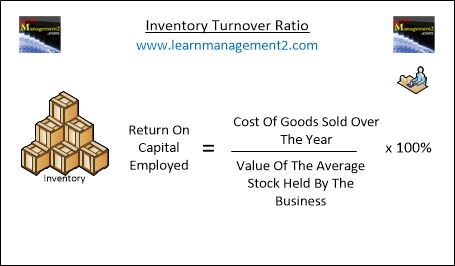# Activity Ratios Overview

Activity ratios analyse what a business is doing with its stock (or inventory), assets and debtors. They are important because they show how a business is performing on a daily basis. There are three activity ratios:

1. Stock Turnover or Inventory Turnover
2. Debtors’ Collection Period
3. Asset Turnover RatioThis diagram shows how to calculate inventory turnover ratio using the cost of goods sold over the year and the value of the average stock held by the business

## Inventory (Stock) Turnover Ratio

This ratio shows how many times a business replaces its stock over the year. The ideal ratio will depend on the type of business. A business selling fresh food will want to sell everything by the end of the day so an ideal ratio for that business is 365. However a car dealership will not expect to sell its entire car stock every day, the ideal stock turnover will depend on the structure and size of the dealership.

### How Do You Calculate Inventory Turnover

The following formula is used to calculate stock turnover ratio:

Stock Turnover Ratio =   Cost of Goods Sold (over the year)

____________________

Value of the average stock held by the business

The answer is expressed as number of times per year. If you are measuring over a different time period you would express your answer as the number of times per that time period e.g. if you were measuring over a month it would be number of times per month.

### Example Inventory Turnover Calculation

In our fictional example Learnmanagement2 own a bookshop called LM2 Books. In 2010 LM2 Books sold books that cost £25000 in total. LM2 Books average stock value is £2500. This means that:

Cost of goods sold = £25000
Average stock value = £2500

To work out the stock turnover ratio for LM2 Books undertake the following calculation:

LM2 Stock turnover ratio =    25000
2500

LM2 Stock turnover ratio =     10

### Calculating Average Inventory (Stock) Value

If you do not know the average inventory (stock) value for the business, add

- the inventory value at the beginning of the year to
- the inventory value at the end of the year

And divide the answer by two. If the inventory value changes monthly add up the monthly stock values for the year together and divide by 12. For example

Month Inventory Value Month Inventory Value
January £39 July £45
February £45 August £66
March £55 September £32
April £49 October £16
May £66 November £59
June £40 December £40

### Average Inventory Value Calculation Answer

To calculate the average inventory value we will need to add up the inventory value for each month and then divide it by 12 as we would like to know the average over a period of 12 months.

Average Inventory Value = £39 + £45+£55+£49+£66+£40+£45+£66+£32+£16+£59+£40

____________________

12

Average Inventory Value = 552/12 = 46

In our example the average inventory value is 12.

We hope you enjoyed learning how to calculate the inventory turnover. Here are some related articles to help you continue learning about activity ratios

Related Articles : Debtor's Collection Period | Asset Turnover Ratio